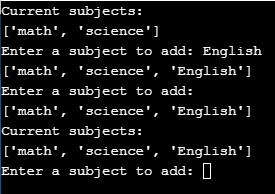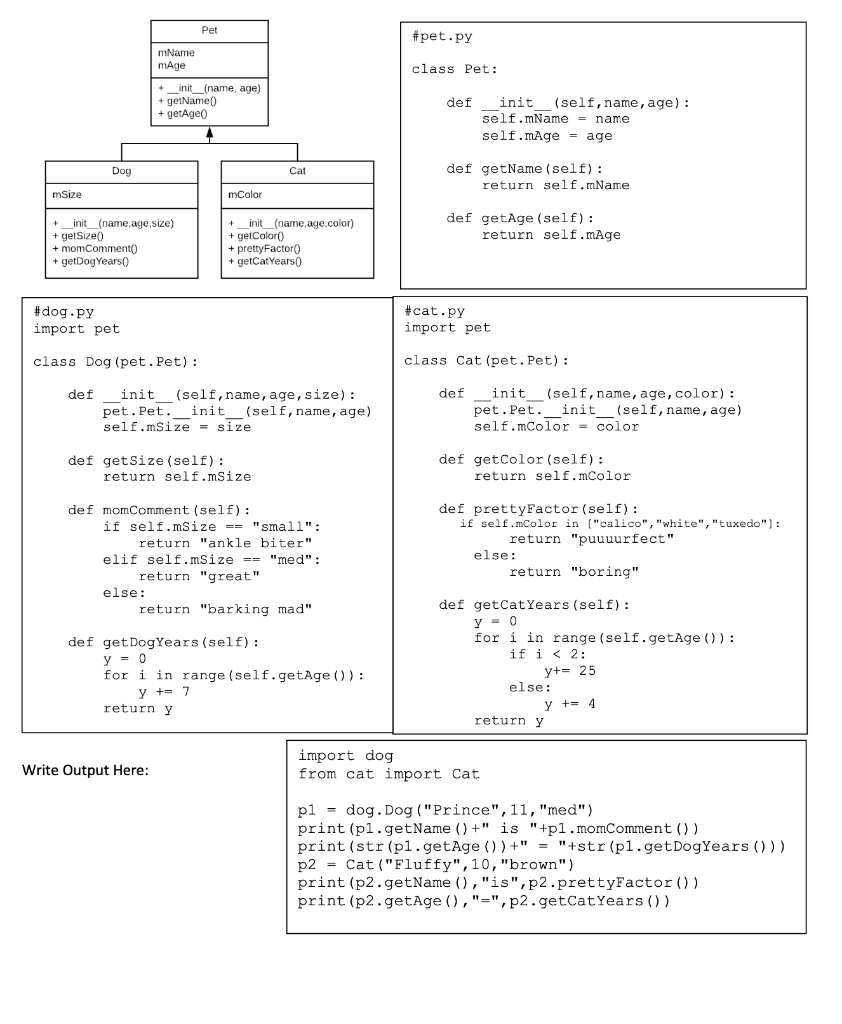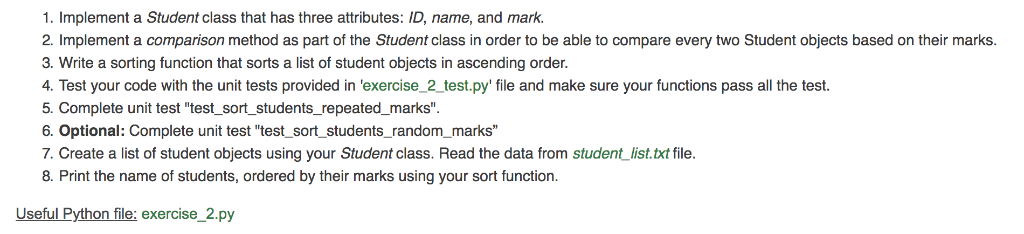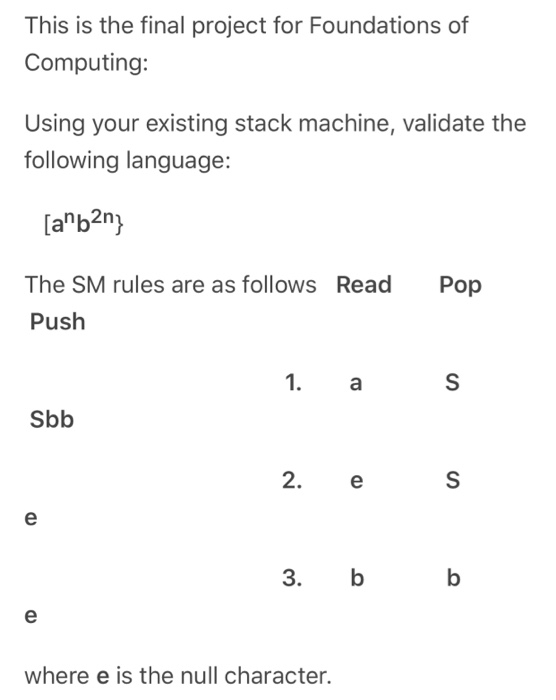Question

# PYTHON 3.8 class student(): def __init__(self, name = 'Bill', grade = 9, subjects = ['math','science']): self.name...

PYTHON 3.8

class student():

def __init__(self, name = 'Bill', grade = 9, subjects = ['math','science']):
self.name = name
self.subjects = subjects

print('Current subjects:')
print(self.subjects)
more_subjects = True
while more_subjects == True:
subject_input = input('Enter a subject to add: ')
if subject_input == '':
more_subjects = False
print(self.subjects)
else:
self.subjects.append(subject_input)
print(self.subjects)

m = student()
d = student()

why is it that when i execute function add_subjects that it adds the subject to both class objects

For proper Explanation let us add two extra line in the code given (Last two lines) to call the function add_subjects() using both the objects m and d.

Code :

class student():
def __init__(self, name = 'Bill', grade = 9, subjects = ['math','science']):
self.name = name
self.subjects = subjects

print('Current subjects:')
print(self.subjects)
more_subjects = True
while more_subjects == True:
subject_input = input('Enter a subject to add: ')
if subject_input == '':
more_subjects = False
print(self.subjects)
else:
self.subjects.append(subject_input)
print(self.subjects)

m = student()
d = student()

Output :Explanation :

Current subjects:

['math', 'science']

Now,it will takes the subject to be added, Here I have added English subjected so it will displays this as following:

Enter a subject to add: English

['math', 'science', 'English']

To get out of m.add_subjects(), we have given an empty value ('') to the subjected to be added, before getting out it will again print the subjects added:

['math', 'science', 'English']

Current subjects:

['math', 'science', 'English']

• Now, it has observed that when we call the d.add_subjects() , the subject we added in m.add_subjects() also appears here, this is because you are calling the same function add_subjects() of the class.
• Also m.add_subjects() has appended the parameters subjects during its execution. Thus when you call the function d.add_subjects(), it will use the subjects list that is appended previously by m.add_subjects().

#### Earn Coins

Coins can be redeemed for fabulous gifts.

Similar Homework Help Questions
• ### Take the class Person. class Person: """Person class""" def __init__(self, lname, fname, addy=''): self._last_name = lname...

Take the class Person. class Person: """Person class""" def __init__(self, lname, fname, addy=''): self._last_name = lname self._first_name = fname self._address = addy def display(self): return self._last_name + ", " + self._first_name + ":" + self._address Implement derived class Student In the constructor Add attribute major, default value 'Computer Science' Add attribute gpa, default value '0.0' Add attribute student_id, not optional Consider all private Override method display() Test your code with the following driver: # Driver my_student = Student(900111111, 'Song', 'River')...

• ### PYTHON -------------------------------------------------------- class LinkedList:    def __init__(self):        self.__head = None        self.__tail = NonePYTHON -------------------------------------------------------- class LinkedList:    def __init__(self):        self.__head = None        self.__tail = None        self.__size = 0    # Return the head element in the list    def getFirst(self):        if self.__size == 0:            return None        else:            return self.__head.element        # Return the last element in the list    def getLast(self):        if self.__size == 0:            return None        else:            return self.__tail.element    # Add an element to the beginning of the list    def addFirst(self, e):        newNode = Node(e) # Create a new node        newNode.next = self.__head # link...

• ### Score Analysis creating a class named "Student" in python and use matplotlib package

class students:       count=0       def __init__(self, name):           self.name = name           self.scores = []           students.count = students.count + 1       def enterScore(self):                     for i in range(4):                 m = int(input("Enter the marks of %s in %d subject: "%(self.name, i+1)))                self.scores.append(m)             def average(self):                 avg=sum(self.scores)/len(self.scores)                           return avg               def highestScore(self):                           return max(self.scores)         def display(self):                          print (self.name, "got ", self.scores)                      print("Average score is ",average(self))                          print("Highest Score among these courses is",higestScore(self))          name = input("Enter the name of Student:")  s = students(name)  s.enterScore()  s.average()  s.highestScore()  s.display()So I need to print two things, first one is to compute the average score of these courses and second one is to print out the course name with the highest score among these courses. Moreover I need to import matplotlib.pyplot as plt to show the score figure. I barely could pull the code this...

• ### 9p This is for Python I need help. Pet #pet.py mName mAge class Pet: + __init__(name,...9p This is for Python I need help. Pet #pet.py mName mAge class Pet: + __init__(name, age) + getName + getAge0 def init (self, name, age): self.mName = name self.mAge = age Dog Cat def getName(self): return self.mName mSize mColor + __init__(name, age,size) + getSize() + momCommento + getDog Years() +_init__(name, age,color) + getColor + pretty Factor + getCatYears def getAge(self): return self.mAge #dog.py import pet #cat.py import pet class Dog (pet. Pet): class Cat (pet. Pet): def init (self,...

• ### Must be in Python 3 exercise_2.py # Define the Student class class Student():    # Ini...Must be in Python 3 exercise_2.py # Define the Student class class Student():    # Initialize the object properties def __init__(self, id, name, mark): # TODO # Print the object as an string def __str__(self): return ' - {}, {}, {}'.format(self.id, self.name, self.mark) # Check if the mark of the input student is greater than the student object # The output is either True or False def is_greater_than(self, another_student): # TODO # Sort the student_list # The output is the sorted...

• ### in python and according to this #Creating class for stack class My_Stack: def __init__(self): self.items = [] def isEmpty(self): return self.items == [] def Push(self, d): self.items.append(d) def Po...in python and according to this #Creating class for stack class My_Stack: def __init__(self): self.items = [] def isEmpty(self): return self.items == [] def Push(self, d): self.items.append(d) def Pop(self): return self.items.pop() def Display(self): for i in reversed(self.items): print(i,end="") print() s = My_Stack() #taking input from user str = input('Enter your string for palindrome checking: ') n= len(str) #Pushing half of the string into stack for i in range(int(n/2)): s.Push(str[i]) print("S",end="") s.Display() s.Display() #for the next half checking the upcoming string...

• ### USE PYTHON CODING ####################### ## ## Definition for the class Pet ## ######################## class Pet: owner=""...

USE PYTHON CODING ####################### ## ## Definition for the class Pet ## ######################## class Pet: owner="" name="" alive=False def __init__(self,new_name): self.name=new_name self.alive=True Using the code shown above as a base write a code to do the following: - Create a separate function (not part of the class) called sameOwner which reports whether two pets have the same owner. test it to be sure it works - Add a variable age to Pet() and assign a random value between 1 and...